# Clocks

How long path will walk the end of the clock's minute-hand long 7 cm for 330 minutes? How long will walk in the same time hour-hand?

Correct result:

minute hand walk:  241.9 cm
minute hand walk:  20.2 cm

#### Solution:

$s_m = 2\pi \cdot \dfrac{ 330}{ 60 } \cdot 7 = 241.9 \ \text{cm}$
$s_h = 2\pi \cdot \dfrac{ 330}{12 \cdot 60} \cdot 7 = 20.2 \ \text{cm}$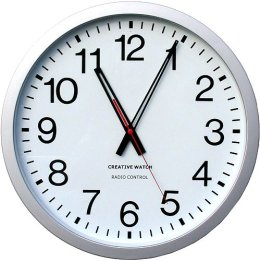We would be pleased if you find an error in the word problem, spelling mistakes, or inaccuracies and send it to us. Thank you!Tips to related online calculators
Do you want to convert length units?
Do you want to convert velocity (speed) units?
Do you want to convert time units like minutes to seconds?

#### You need to know the following knowledge to solve this word math problem:

We encourage you to watch this tutorial video on this math problem:

## Next similar math problems:

• Acceleration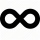Describe how the cyclist's acceleration changes on individual sections (sections AB plane, BC turn, CD plane, DA turn), which describes the trajectory in the shape of an eight at a constant speed. The speed on the cyclist's tachometer is constant
• RPMAn electric motor makes 3,000 revolutions per minutes. How many degrees does it rotate in one second?
• Velocipedes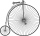In the 19th century, bicycles did not have a chain drive, and the pedals were connected directly to the wheel axis. This wheel diameter gradually increased until the so-called high bicycles (velocipedes) with a front wheel diameter of up to 1.5 meters, wh
• A large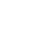A large gear will be used to turn a smaller gear. The large gear will make 75 revolutions per minute. The smaller gear must make 384 revolutions per minute. Find the smallest number of teeth each gear could have. [Hint: Use either GCF or LCM. ]
• Two gears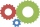The two gears fit together. The larger gear has 32 teeth, the smaller has 20 teeth less. How many times does turn a smaller gear if the bigger gear turns three times?
• Earth's diameter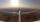The Earth's diameter on the equator is approximately 12750 km. How long does the Gripen flyover the Earth above the equator at 10 km if it is at an average speed of 1500 km / h?
• Average speed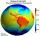What is the average speed you have to move the way around the world in 80 days? (Path along the equator, round to km/h).
• FlywheelFlywheel turns 450 rev/min (RPM). Determine the magnitude of the normal acceleration of the flywheel point which are at a distance of 10 cm from the rotation axis.
• Ferris wheel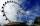Ferris wheel reaches to 22 m tall and moves at the speed of 0.5m/s. During one drive wheel rotates three times. What is the total drive time?
• Velocity ratio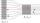Determine the ratio at which the fluid velocity in different parts of the pipeline (one part has a diameter of 5 cm and the other has a diameter of 3 cm), when you know that at every point of the liquid is the product of the area of tube [S] and the fluid
• Clock Tower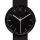What angle is betwenn hands on Clock Tower when show 17 hours and 35 minutes?
• Saw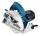Blade circular saw with a diameter 42 cm turns 825 times per minute. Expresses his cutting speed in meters per minute.
• Circular motionMass point moves moves uniformly in a circle with radius r = 3.4 m angular velocity ω = 3.6 rad/s. Calculate the period, frequency, and the centripetal acceleration of this movement.
• Trough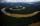How many liters of water per second can go via trough, which has a cross section of semicircle with radius 2.5 m and speed of water is 147 cm per second?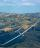Calculate how many g's (gravity accelerations) feel glider pilot when turning the horizontal circles of radius 148 m flying at 95 km/h. Centripetal acceleration is proportional to the square of the speed and inversely proportional to the radius of rotatioHow many times a day hands on a clock overlap?The clock shows 12 hours. After how many minutes will angle between the hour and minute hand 90°? Consider the continuous movement of both hands hours.